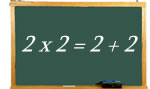Logic puzzles, riddles, math puzzles and brainteasers - pzzls.com

Vandaag is het 10 August 2022

Sort - Easiest on top
Page: << 1 2 3 4 5 >>

# Let's go for a hike - math puzzle

Difficulty:Rating: 2.8/5.0

A hiker walked for two days. On the second day the hiker walked 2 hours longer and at an average speed 1 mph faster than he walked on the first day. If during the two days he walked a total of 64 miles and spent a total of 18 hours walking, what was his average speed on the first day?

# Letter folding - math puzzle

Difficulty:Rating: 2.6/5.0

If you would fold a letter in two fifty times, how thick should the enveloppe be that can fit the letter? Assume that the thickness of a piece of paper is about 0.05 mm.

# 2 x 2 = 2 + 2 - math puzzle

Difficulty:Rating: 2.9/5.0

 Two times two is equal to two and two. But are there more number pairs for which the sum of the numbers is is equal to the product of the numbers? The numbers may be different.